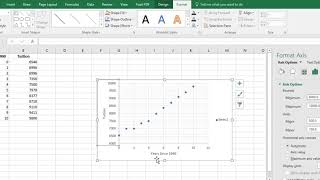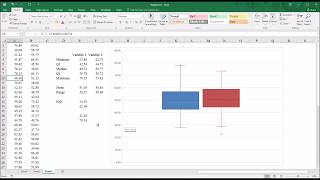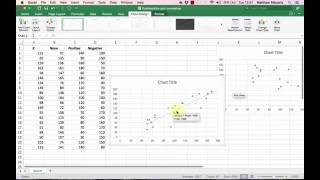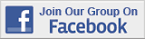Excel Tips & Tutorials:
Graphing Relationships and Describing Patterns
 Suggest a Link Alphabetize Page Printer-Friendly List
It Has to Start Sometime
Watch and Learn
Watch and Learn
Watch and Learn
Watch and Learn
 Creating a Scatter PlotShows you how to make a basic scatterplot and edit it for clarity (4:38).Creating a BoxplotTutorial from Todd Grande (12:03).Working with Different Chart TypesDemonstrated with book sales data (4:30).How to Make and Interpret a ScatterplotFrom Matt Macarty (7:08).Creating a Scatter PlotShows you how to make a basic scatterplot and edit it for clarity (4:38).Creating a BoxplotTutorial from Todd Grande (12:03).Working with Different Chart TypesDemonstrated with book sales data (4:30).Creating a Scatter PlotShows you how to make a basic scatterplot and edit it for clarity (4:38).Creating a BoxplotTutorial from Todd Grande (12:03).Creating a Scatter PlotShows you how to make a basic scatterplot and edit it for clarity (4:38).Over 18,000 Members!